# The MCMC Procedure

### Example 73.11 Nonignorably Missing Data (MNAR) Analysis

This example illustrates how to fit a nonignorably missing data model (MNAR) with PROC MCMC. For a short overview of missing data problems, see the section Handling of Missing Data.

This data set comes from an environmental study that involve workers in a cotton factory. A similar data set was analyzed from Ibrahim, Chen, and Lipsitz (2001). There are 912 workers in the data set, and the response variable of interest is whether they develop dyspnea (difficult or labored respiration). The data are collected over three time points, and there are six covariates. The following statements create the data set:

title 'Nonignorably Missing Data Analysis';
data dyspnea;
input smoke1 smoke2 smoke3 y1 y2 y3 yrswrk1 yrswrk2 yrswrk3
age expd sex hgt;
datalines;
0  0  0  0  0  0  28.1  33.1  39.1  48 1 1 165.0
0  0  0  0  .  0   5.1  10.1  16.1  45 1 0 147.0
0  0  0  0  .  0  26.0  31.0  37.0  46 1 0 156.0

... more lines ...

1  1  1  0  .  .   6.0  11.0  17.0  25 0 1 180.0
0  0  0  0  .  .  20.0  25.0  31.0  42 0 0 159.0
;


The following variables are included in the data set:

• y1, y2, and y3: dichotomous outcome at the three time periods, which takes the value 1 if the worker has dyspnea, 0 if not (there are missing values in y2 and y3)

• smoke1, smoke2, smoke3: smoking status (0=no, and 1=yes)

• yrswrk1, yrswrk2, yrswrk3: years worked at the cotton factory

• age: age of the worker

• expd: cotton dust exposure (0=no, 1=yes)

• sex: gender (0=female, 1=male)

• hgt: height of the worker

Prior to the analysis, three missing data indicator variables (r1, r2, and r3, one for each of the response variables) are created, and they are set to 1 if the response variable is missing, and 0 otherwise. The covariates age, hgt, yrswrk1, yrswkr2, and yrswrk3 are standardized:

data dyspnea;
array y y1-y3;
array r;
set dyspnea;
do i = 1 to 3;
if y[i] = . then r[i] = 1;
else r[i] = 0;
end;
output;
run;

proc standard data=dyspnea out=dyspnea mean=0 std=1;
var age hgt yrswrk:;
run;


There are no missing values in response variable y1, 128 missing values in y2, and 131 in y3. Ibrahim, Chen, and Lipsitz (2001) used a logistic regression for each of the response variables, where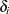is a scalar random effect on the observational level: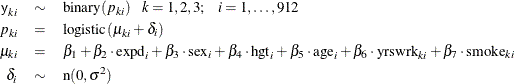Ibrahim, Chen, and Lipsitz (2001) noted that takingto be higher dimensional (3) would make the model either not identifiable or nearly not identifiable because of the multiple missing values for some subjects.

The first response variable y1 does not contain any missing values, making it meaningless to model the corresponding r1 because every value is 1. Hence, only r2 and r3 are considered in the missing mechanism part of the model. Ibrahim, Chen, and Lipsitz (2001) suggest the following logistic regression for r2 and r3, where the regression mean for each r depends not only on the current response variable y but also the response from previous time period: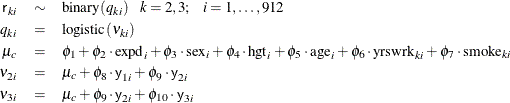The missing mechanism model introduces an additional 10 parameters to the model. Normal priors with large standard deviations are used here.

The following statements fit a nonignorably missing model to the dyspnea data set:

ods select MissDataInfo REParameters Postsumint;
proc mcmc data=dyspnea seed=17 outpost=dysp2 nmc=20000
propcov=simplex diag=none monitor=(beta1-beta7);
array p;
array yrswrk;
array smoke;

parms beta1-beta7 s2;
parms phi1-phi10;
prior beta: phi: ~ n(0, var=1e6);
prior s2 ~ igamma(2, scale=2);
random d ~ n(0, var=s2) subject=_obs_;
mu = beta1 + beta2*expd + beta3*sex + beta4*hgt + beta5*age + d;
do j = 1 to 3;
p[j] = logistic(mu + beta6*yrswrk[j] + beta7*smoke[j]);
end;
model y1 ~ binary(p1);
model y2 ~ binary(p2);
model y3 ~ binary(p3);

nu = phi1 + phi2*expd + phi3*sex + phi4*hgt + phi5*age;
q2 = logistic(nu + phi6*yrswrk + phi7*smoke +
phi8*y1 + phi9*y2);
model r2 ~ binary(q2);
q3 = logistic(nu + phi6*yrswrk + phi7*smoke +
phi9*y2 + phi10*y3);
model r3 ~ binary(q3);
run;


The first ARRAY statement declares an array p of size 3. This arrays stores three binary probabilities of the response variables. The next two ARRAY statements create storage arrays for some of yrswrk and smoke variables for later programming convenience. The first PARMS statement declares eight parameters,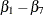and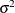. The second PARMS statement declares the 10parameters for the missing mechanism model. The PRIOR statements assign prior distributions to these parameters.

The RANDOM statement defines an observational-level random effect d that has a normal prior with variance s2. The SUBJECT=_OBS_ option enables the specification of individual random effects without an explicit input data set variable.

The MU assignment statement and the following DO loop statements calculate the binary probabilities for the three response variables. Note that different yrswrk and smoke variables are used in the DO loop for different years. The three MODEL statements assign three binary distributions to the response variables.

The NU assignment statement starts the calculation for the regression mean in the logistic model for r2 and r3. The variables q2 and q3 are the binary probabilities for the missing mechanisms. Note that their calculations are conditional on the response variables y (pattern mixture model). The last two MODEL statements for r2 and r3 complete the specification of the models.

Missing data information and random-effects parameters information are displayed in Output 73.11.1. You can read the total number of missing observations from each variable and its indices from the table. The missing values are sampled using the inverse CDF method. There are 912 random-effects parameters in the model.

Output 73.11.1: Missing Data and Random-Effects Information

 Nonignorably Missing Data Analysis

The MCMC Procedure

Missing Data Information Table
Variable Number of
Missing Obs
Observation
Indices
Sampling
Method
y2 128 2 3 9 11 13 19 20 21 30 31 32 35 39 40 43 56 58 71 75 95 ... Inverse CDF
y3 131 9 14 16 20 21 29 31 32 43 45 56 72 86 115 117 121 124 142 149 160 ... Inverse CDF

Random Effect Parameters
Parameter Sampling
Method
Subject Number of
Subjects
Subject
Values
Prior
Distribution
d N-Metropolis _OBS_ 912 1 2 3 4 5 6 7 8 9 10 11 12 13 14 15 16 17 18 19 20 ... normal(0, var=s2)

The posterior summary and interval statistics of all the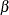parameters are shown in Output 73.11.2. There are a number of significant regression coefficients in modeling the probability of a worker developing dyspnea, including those for expd (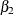), sex (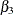), age (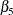), and smoke (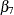).

Output 73.11.2: Posterior Summary Statistics forNonignorably Missing Data Analysis

The MCMC Procedure

Posterior Summaries and Intervals
Parameter N Mean Standard
Deviation
95% HPD Interval
beta1 20000 -2.3256 0.1771 -2.6670 -1.9826
beta2 20000 0.5327 0.1530 0.2306 0.8193
beta3 20000 -0.5966 0.2593 -1.0906 -0.0691
beta4 20000 -0.0682 0.1061 -0.2734 0.1462
beta5 20000 0.6252 0.1640 0.2992 0.9490
beta6 20000 -0.1776 0.1574 -0.4971 0.1218
beta7 20000 0.5862 0.2214 0.1433 1.0051